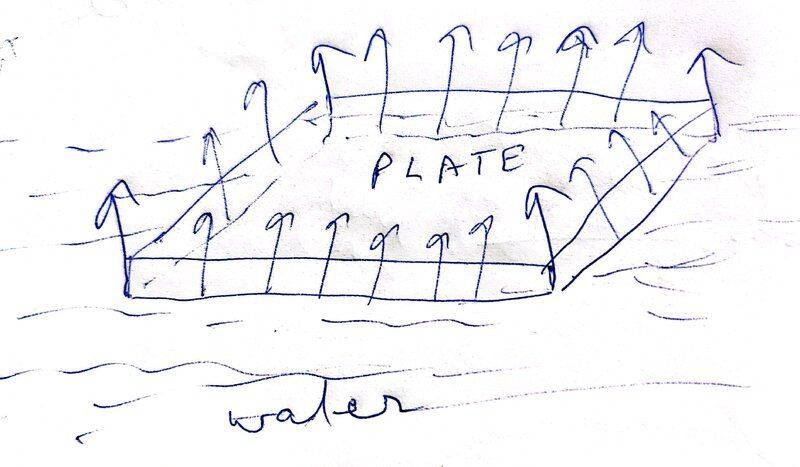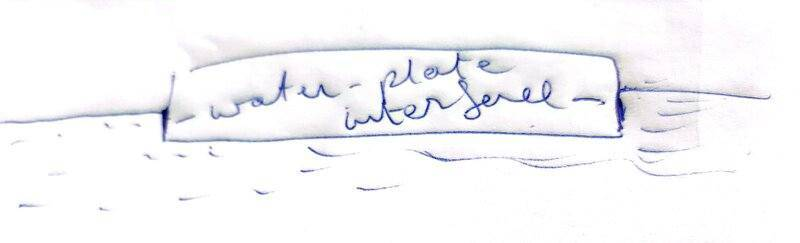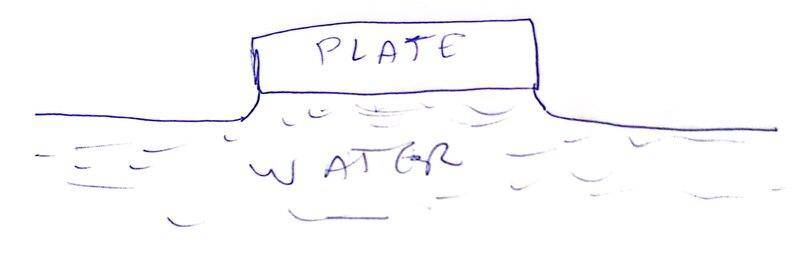# Surface Tension: Plate-Water Interface

• vcsharp2003

#### vcsharp2003

Homework Statement
A rectangular plate of dimensions 6 cm by 4 cm and thickness 2 mm is placed with its largest face flat on the surface of water. Calculate the force due to surface tension on the plate. What is the downward force due to surface tension if the plate is placed vertical and its longest side just touches the water? (Surface tension of water = 7000 N/m.)
Relevant Equations
##S= \frac {F}{l}##, where ##S## is the surface tension perpendicular to an imaginary line on the liquid's surface of length ##l## on which a force of ##F## acts due to surface tension ( The force ##F## lies in the plane of the interface between liquid and another material)
I would think that a force due to surface tension would act upwards on the plate and not downward, as shown in the diagram I have come up with. The surface of water would act as a stretched membrane and therefore there would be a surface tension on water surface in contact with the bottom of the plate edges and it would act along the area of contact created at the edges.

The interface between water and plate would be as shown in second diagram, which supports the direction of surface tension in first diagram since we can consider the imaginary line on water surface coinciding with the plate edge and then the liquid surface which extends vertically up the edge face of plate would pull this imaginary line upwards.In the second part, they mean with some external force holding up the plate such that if the force were increased the plate would lose contact with the water .

•vcsharp2003
In the second part, they mean with some external force holding up the plate such that if the force were increased the plate would lose contact with the water .
So the meniscus between plate and water surface is assumed to be concave i.e. angle of contact is acute and we idealize by assuming angle of contact is 0 degrees i.e. the liquid surface is vertical next to each edge else the surface tension would not point vertically downwards. ( As shown in diagram below)

Is the above scenario what we need to assume for this situation?Last edited:
yes

•vcsharp2003
yes
I hope these questions were given with more clarity. But I guess, making assumptions is part of the process of problem solving in Physics.